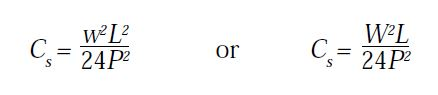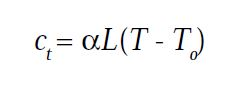# Errors in Chain Surveying

Every measurement made with a mechanical device is subject to any error that could possibly be caused by the condition of the device or by the procedure used in taking the measurement.

Some of the more common sources of errors are the

• standards to which the chain was manufactured,
• any damage to the chain, sag in the chain,
• variation in the tension on the chain, and
• changes in the temperature of the chain.

With proper care of the chain and reasonable effort made with each use, the effects of these errors can be kept within acceptable tolerances for all but the most precise measurements.

When necessary, each of these conditions can be compensated for mathematically if they are monitored and compared to a known standard.

## 1. Proportional Errors

When a chain is manufactured, it is intended to be a specific length, plus or minus some tolerance. It may or may not actually meet those specifications. When a field measurement is taken, the acceptable error may be more or less than what the chain was designed for.

For high precision work, we need to measure several known distances and determine if this chain is the proper length. If not, we need next to determine if the error is in one or more specific locations along the chain or if the error is proportional along the length.

If a known 50 foot distance is measured to be 49.995 feet and a known 100 foot distance to be 99.99 feet, all measurements made with that chain should be multiplied by a factor of 100/ 99.99 (known distance over measured distance).

## 2. Constant Errors

If a chain has been kinked or broken and spliced back together, there is a good chance that there will be a consistent error for any distances measured using that portion of the chain.

This error needs to be added or subtracted as appropriate each time.

## 3. Sag Correction

When a chain is suspended from each end and not supported along its length, the weight of the chain causes it to sag and pulls the two ends toward each other. It is impossible to exert enough outward force to fully overcome the sag.

For all measurements, adequate tension should be applied to minimize the effective shortening of the chain. For precise measurements, a correction should be applied using the formula given below.where:

C s = Sag Correction between points of support, (Ft.)

w = Weight of tape, (Lb.s / Ft.)

W = Total Weight of tape between supports, (Lb.s)

L = Distance between supports (Ft.)

P = Applied Tension, (Lb.s)

## 4. Tension Correction

While a certain amount of tension is desirable to help offset the sag effect, it will also stretch the chain. Steel is generally thought of as not being very easily stretched and indeed it is not. That is one of the reasons it is used for making chains. But steel will still stretch to some degree if tension is applied.

When a chain is checked against a known distance, the applied tension should be controlled. Subsequent precise measurements should be made using the same tension, or if not, a correction should be applied. The formula for this is also mentioned below.where:

Cp = Correction per distance L

P = Applied Tension (Lb.s)

P0 = Tension for which the tape was standardized.

L = Length, (Ft.)

a = Cross-Sectional Area of the Chain.

E = Modulus of Elasticity of Steel. (30 * 106 lb/in2)

## 5. Temperature Correction

Whatever material is used to make a chain, that material will expand and contract with any change in temperature. Some materials are more affected than others, but every chain will change length somewhat if warmed or cooled.

If precise measurements are needed, an adjustment needs to be made for the change in temperature between the current temperature and the temperature at the time the chain was checked against a known distance. This formula is also given below.where:

α = Coefficient of thermal expansion (0.00000645 / 1o F)

L = Measured Length

T = Temperature of Chain

T = Standard Temperature ( 68o F )